Courses

# Flow Dynamics in Open Channels and Rivers (Part - 1) Civil Engineering (CE) Notes | EduRev

## Civil Engineering (CE) : Flow Dynamics in Open Channels and Rivers (Part - 1) Civil Engineering (CE) Notes | EduRev

The document Flow Dynamics in Open Channels and Rivers (Part - 1) Civil Engineering (CE) Notes | EduRev is a part of Civil Engineering (CE) category.
All you need of Civil Engineering (CE) at this link: Civil Engineering (CE)

Introduction

It is common for water resources engineers to design a water system involving flow of water from one place to another, usually passing a variety of structures on the way some of them meant for controlling the flow quantity. Rivers and artificial channels, like canals, convey water with a free surface, that is, the surface of water being exposed to air as opposed to flow of water in pipes. It is easy to visualize that for any such open channel flow, as they are called; the presence or absence of a hydraulic structure controls the position of the free surface of water. Knowing the mathematical description of flowing water, it is possible to compute the water surface profile, which is important for example in designing the height of the channel walls of the water conveying system.

Another example, the case of river flow obstruction by the presence dam may be mentioned. The water level of the river increases on construction of the dam and it is essential to know the maximum possible rise, perhaps during the maximum flood, in order to know the degree of submergence of the land behind the dam. Barrages are low height structures, and hence, the rise of water will not be occurring uniformly across the river, again due to the difference of gate operation.

In this lesson, the behavior and corresponding mathematical description of flow in open channels are reviewed in order to utilize them in designing water resources systems.

Flow in natural rivers

Figure1 shows a river carrying a low discharge.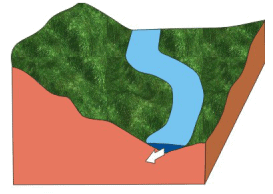FIGURE, 1 A river flowing within its banks .

When the water surface of the river just touches its banks, the discharge flowing through the river at this stage is called the “bank full discharge”. It is also sometimes called the “dominant discharge”. If the discharge in the river increases, the water will overflow the banks and would spill over to the adjacent land, called the flood plains (Figure 2).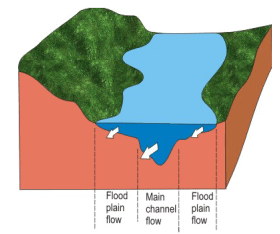FIGURE 2 A river flowing its banks during flood

Though the amount of discharge flowing through the river is of interest to the water resources engineer it cannot be measured directly by any instruments. Rather, an indirect method is used which requires knowledge of the velocity distribution in a river or an open channel.

If we plot the velocity profile across a river, as shown in Figure 1, it would actually vary in three dimensions. Figure 3 shows the variation of velocity at the water surface.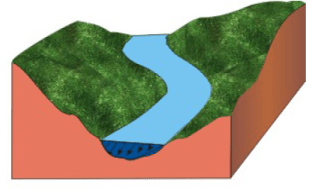FIGURE 3 . Variation of surface velocity across a river section

It may be observed that velocity is highest at the center of the river but is zero at the banks. If a velocity profile were plotted on another horizontal plane at certain depth of the river, there too the velocity profile would be found to be similar in shape, but smaller in magnitude (Figure 4).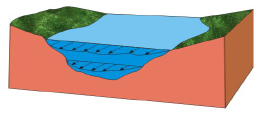FIGURE 4 . Velocity variation of a river section at two tevels.

Similarly the velocity profile of the river flowing in flood would be as shown in Figure 5, showing that the velocities over the flood plains is smaller compared to the main stream flow.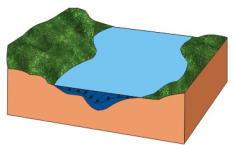FIGURE 5. Surface velocity profile across a river section for a river flowing in flood

If we now take a look at the variation of velocity in a vertical plane within a river, and we plot them along different vertical lines across the river, then we may find the velocity profiles similar to those shown in Figure 6.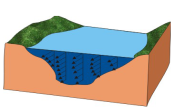FIGURE 6. Vertical velocity profiles in a river section

In order to measure the discharge being conveyed in a river, the velocity profile or the average velocity at a number of equally spaced sections are measured, as in Figure 6. The total discharge is then approximately taken equal to the sum of the discharges passing through each segment.

Another way of depicting the velocity variation across a river cross-section is to plot “Isovels”, which are actually the locus of points having equal velocity (Figure 7).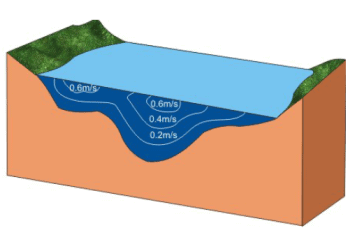FIGURE 7. Isovels showing contours of typical equal velocities across a typical river section

It has been observed through experiments that a plot of velocity in the vertical plane would show that the maximum velocity occurs slightly below the surface (Figure 8) for a typical river flow.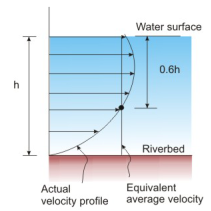FIGURE 8. Vertical velocity distribution and average velocity for flow in a river

It has further been observed that an equivalent average velocity is almost equal to the actual velocity measured at 0.6 depth.

Variation of discharge with river stage

The water level in a river is sometimes called the “stage” and as this varies, there is a proportional change in the total discharge conveyed. For each point of a river, the relation between stage and discharge is unique but a general form is found to be as shown in Figure 9.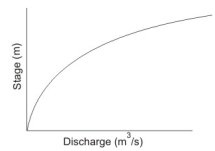FIGURE 9. Stage-discharge curve for a river

The general mathematical description for the stage-discharge relation is given as:

Q =  k ( h - h0 )m         (1)

Where h is the gauge corresponding to a discharge Q and h0 is the corresponding to zero discharge k and m are constants. If the variables (Q and H) are plotted on a log-log graph, then it generally plots in a straight line as:

log Q = m log (h - h0 ) + log k             (2)

Flow variation along river length

It may be interpreted from Figures 4 or 6 that the velocity in a river cross section actually varies from bank to bank and from riverbed to free water surface and hence, can be called a two dimensional variation in a vertical plane. However, for engineering purposes it is, sufficient, generally, to use an equivalent velocity in the direction of river motion (perpendicular to river cross section) which may be obtained by dividing the total discharge by the cross sectional area. In a natural river, therefore, these flow velocities may vary from section to section (Figure 10).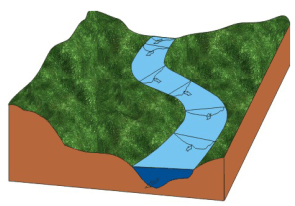FIGURE. 10:- Variation of cross section and velocity along the length of a river showing vertical section at each point

If we now consider an axis along the length of the river, the total energy (H) is given as:

H = Z + h + V2 / 2g               (3)

We may plot the total energy as shown in Figure 11, where the variables are as follows:

• Z: Height of riverbed above a datum
• h: Depth of water
• V: Average velocity at a section
• V2 / 2g : Kinetic energy head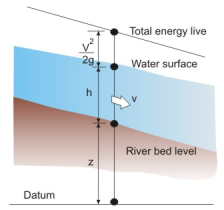FIGURE 11. Variation of total energy along a river

Since the cross section, bed slope and flow resistance vary along a river length, the depth and velocity would vary correspondingly. However, if a short stretch of a river section is taken, then the variations in riverbed, water surface and the total energy may be considered as linear (Figure 12).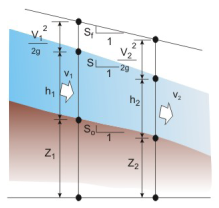FIGURE 12. Short section between two points along a river

In Figure 12, three slopes have been marked, which are:

• S0: Riverbed slope
• S: Water surface slope
• Sf: Energy surface slope

Since the total energy of flowing water reduces along the river length due to friction the “energy surface slope” is generally termed as the “friction slope”. The energy loss in a river or an open channel occurs mostly due to the resistance at the channel sides, as the turbulent characteristics of the flowing water implies a smaller loss internally within the water body itself.

It has been nearly 200 years when scientists first attempted to mathematically express (or “model”) the friction slope in terms of known variables like average velocity, cross section properties and riverbed slope. One of the earliest models for friction slope Sf or, in effect, the channel resistance was derived from the considerations of “uniform flow” (Figure 13) where the flow variables and cross section are supposed to remain constant over a short reach.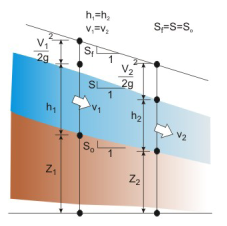FIGURE 13. Uniform flow along a channel reach

If we take small volume of fluid from these two sections we may make a free body diagram of the forces acting on it (Figure 14).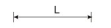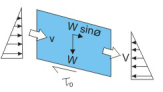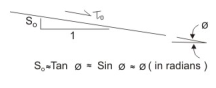FIGURE 14. Free body diagram of forces on a control volume in uniform flow

The variables represented in the figure are as follows

• W: Weight of water contained in the control volume
• V: Inflow velocity, which is the same as the outflow velocities
• θ: Angle of slope river bed, which is also equal to that water surface and friction slopes
• τ 0 : Shear stress due to friction acting on the control volume of fluid from the river bed and all along the periphery, though in Figure 14 only the resistance due to the riverbed is shown.

Equating the forces and noting that the inflowing and out flowing momenta are equal as well as the pressure forces at either end of the control volume one obtains:

τ0 P L = W sinθ = ρ g A L sinθ            (4)

Where the remaining variables are:

• P: wetted perimeter
• A: Cross section of flow area
• L: Length of control volume

Assuming θ to be very small and nearly equal to bed slope, we have

τ0 = ρ g R S0                (5)

Assuming a state of rough turbulent flow, as is the case for natural rivers and channels, one may write

τ0 α V2     or       τ0 = kV2

Substituting into (4),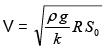(7)

This may be written as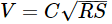(8)

This is known as Chezy equation after the French hydraulic engineer. Antoine Chezy who first proposed the formula around 1768 while designing a canal for Paris water supply. The constant C in equation (8) actually varies depending on Reynolds number and boundary roughness.

In 1869, Swiss engineers, Ganguillet and Kutter proposed an elaborate formula for Chezy’s C which they derived from actual discharge data from the river Mississippi and a wide range of natural and artificial channels in Europe. The formula, in metric units, is given as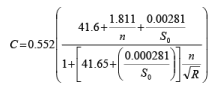(9)

Where n is a coefficient known as Kutter’s n, and is dependent solely on the boundary roughness.

In 1889, Robert Manning’s, an Irish engineer proposed another formula for the evaluation of the Chezy coefficient, which was later simplified to: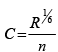(10)

From Equation (8), the Manning equation may be written as: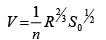(11)

Where the Manning n is numerically equivalent to Kutter’s n.

Many research workers have experimentally found the value of n, and for natural rivers, the following books may be consulted:

1. Chow, V T (1959) “Open Channel Hydraulics”, McGraw Hill.
2. Chaudhry, M H (1994) “Open Channel Flow”, Prentice Hall of India.
Offer running on EduRev: Apply code STAYHOME200 to get INR 200 off on our premium plan EduRev Infinity!

,

,

,

,

,

,

,

,

,

,

,

,

,

,

,

,

,

,

,

,

,

;